Basic probability

Basic Probability Definition

What is probability theory? A branch of mathematics concerned with the analysis of random phenomena. The outcome of a random event cannot be determined before it occurs, but it may be any one of several possible outcomes. The actual outcome is considered to be determined by chance.

Fundamental Terms

2.

Continuous Random Variable is one which takes an infinite number of possible values. Continuous random variables are usually measurements.

A simple example is the tossing of a fair (unbiased) coin. Since the coin is fair, the two outcomes (“heads” and “tails”) are both equally probable; the probability of “heads” equals the probability of “tails”; and since no other outcomes are possible, the probability of either “heads” or “tails” is 1/2 (which could also be written as 0.5 or 50%).

Basic Probability Formulas

0 ≤ P(A) ≤ 1

Rule of Complementary Events

P(AC) + P(A) = 1

P(A∪B) = P(A) + P(B) - P(A∩B)

Disjoint Events

Events A and B are disjoint iff

P(A∩B) = 0

Conditional Probability

P(A | B) = P(A∩B) / P(B)

Bayes Formula

P(A | B) = P(B | A) ⋅ P(A) / P(B)

Independent Events

Events A and B are independent iff

P(A∩B) = P(A) ⋅ P(B)

FX(x) = P(Xx)

Probability Mass Function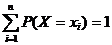Probability Density Function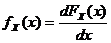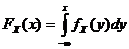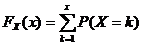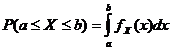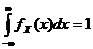Covariance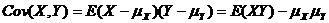Correlation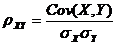Bernoulli: 0-failure 1-success

Geometric: 0-failure 1-success

Hypergeometric: N objects with K success objects, n objects are taken.

Currently, we have around 1977 calculators, conversion tables and usefull online tools and software features for students, teaching and teachers, designers and simply for everyone.